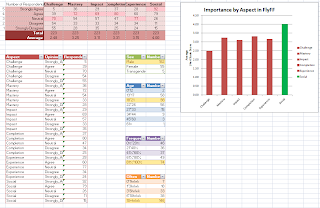## Monday, November 28, 2011

### Active Learning for Defect Data

Here is a nice report about the active learning experiments.

## Monday, November 14, 2011

### Replayability Surveys

Survey results for a few types of games:

MMO RPG Game (FlyFF):Board games:

1. Bias Variance Analysis
a) Anova -> Bias is 8/20, Variance is 14/20
b) Scott&Anova -> Bias is 8/20, Variance is 15/20
c) Anova plus Cohen's correction: Bias is 8/20
d) Kruskal-Wallis not so good: Bias is 4/20 and variance is 10/20

e) Sorted bias values of all the data sets plotted are here.
The above plots showed us that there are extreme values due to new experimental conditions.
f) The Friedman-Nemenyi plots are here for separate data sets.
g) The same test as before for the entire 20 data sets together are here.
h) Re-generate boxplots with the new experimental conditions.

I applied a correction on the analysis, because...
Here is the paper with new boxplots..
Here is the Friedman Nimenyi plots on separate data sets, bias is the same for 15 out of 20 data sets..
Below is the result of Friedman Nimenyi over all data sets, i.e. if we see it as 20 repetitions.

2. Papers
a) HK paper is revised and is ready to be seen here.
b) Active learning paper's major revision is submitted to TSE.
c) Camera ready versions of ensemble and kernel papers are submitted to TSE and ESEM.

3. The break-point experiments

### Clustering Accelerates NSGA-II

Using a natural clustering method, and replacing poor solutions with solutions bred within "interesting" clusters, appears to accelerate how quickly NSGA-II converges upon the Pareto frontier with little impact on the diversity of the solutions or the speed of the algorithm.

Clusters are divided by heuristically and recursively dividing space along a natural axis for the individuals. The FastMap heuristic is used to pick individuals that are very distant from each other by their independent attributes. All the other individuals are placed in the coordinate space by the magnitude of their orthogonal projections onto the line between the two extreme individuals. A division point is chosen along this axis that minimizes the weighted variance of the individuals' ranks. The splitting continues on each half of the split down to "sqrt(individuals)".

An "interesting" cluster is one that has at least one first-rank individual within it and a low ratio of first-rank individuals to total individuals in the cluster. A "boring" cluster is either completely saturated with first-rank individuals or lower-ranked individuals.

## Tuesday, November 1, 2011

### Raw data from hall et al

A Systematic Review of Fault Prediction Performance in Software Engineering

### stats methids

divide numbers such that the entropy of the treatments they select is minimal: http://unbox.org/stuff/var/timm/11/id/html/entropy.html divide performancescores such that the variance t is minimal: http://unbox.org/stuff/var/timm/11/id/html/variance.html
```given N  arrays of performance results p .... of treatments 1,2,3...
with means  m,m, etc
with stand devs of s, s, etc then ...

for i in N
for j in N
boring[i,j] = (abs(m[i] - m[i])/s <= 0.3) #cohen

samples=1000
N*samples timesRepeat: {
i = any index 1..N
j= any index 1..N
if (boring[i,j] continue
x = the i-th member of p[i]
y = the j-th member of p[j]
diff = x - y
better = if(errorMeasures) diff<0 else diff>0
win[i] += better
}
for(i in win)
win[i] = win[i]/(N*samples) * 100 # so now its percents

rank treatments by their position in win (higher rank = more wins)
```